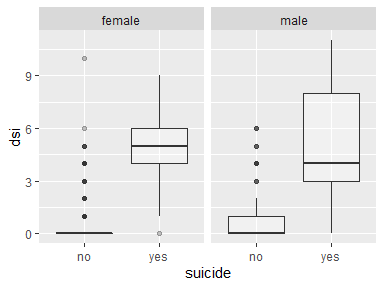# Plotting with cutpointr

#### 2022-04-13

cutpointr includes several convenience functions for plotting data from a cutpointr object. These include:

• plot_cutpointr: General purpose plotting function for cutpointr or roc_cutpointr objects
• plot_cut_boot: Plot the bootstrapped distribution of optimal cutpoints
• plot_metric: If maximize_metric or minimize_metric was used this function plots all possible cutoffs on the x-axis vs. the respective metric values on the y-axis. If bootstrapping was run, a confidence interval based on the bootstrapped distribution of metric values at each cutpoint can be displayed. To display no confidence interval set conf_lvl = 0.
• plot_metric_boot: Plot the distribution of out-of-bag metric values
• plot_precision_recall: Plot the precision recall curve
• plot_sensitivity_specificity: Plot all cutpoints vs. sensitivity and specificity
• plot_roc: Plot the ROC curve
• plot_x: Plot the distribution of the predictor variable
library(cutpointr)

set.seed(123)
opt_cut_b_g <- cutpointr(suicide, dsi, suicide, gender, boot_runs = 500)
## Assuming the positive class is yes
## Assuming the positive class has higher x values
## Running bootstrap...
plot_cut_boot(opt_cut_b_g)
## Warning: Removed 1 rows containing non-finite values (stat_density).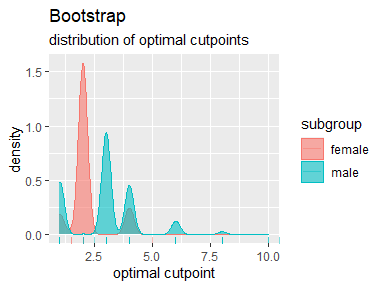plot_metric(opt_cut_b_g, conf_lvl = 0.9)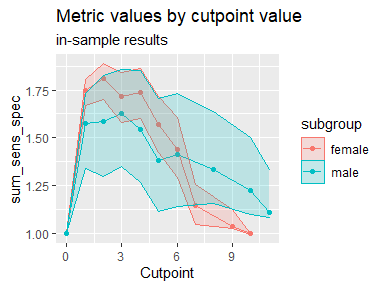plot_metric_boot(opt_cut_b_g)
## Warning: Removed 8 rows containing non-finite values (stat_density).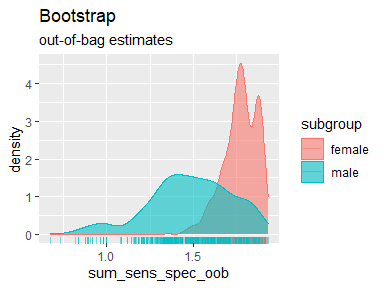plot_precision_recall(opt_cut_b_g)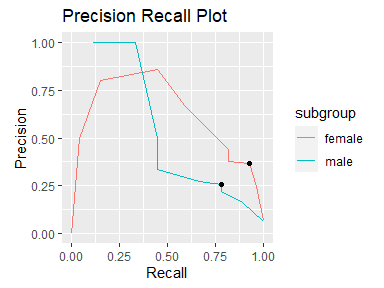plot_sensitivity_specificity(opt_cut_b_g)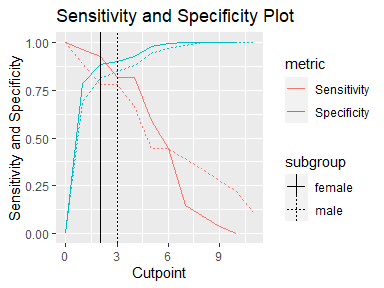plot_roc(opt_cut_b_g)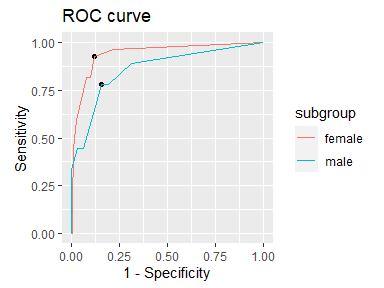All plot functions, except for the standard plot method that returns a composed plot, return ggplot objects than can be further modified. For example, changing labels, title, and the theme can be achieved this way:

library(ggplot2)
p <- plot_x(opt_cut_b_g)
p + ggtitle("Distribution of dsi") +
theme_minimal() +
xlab("Depression score")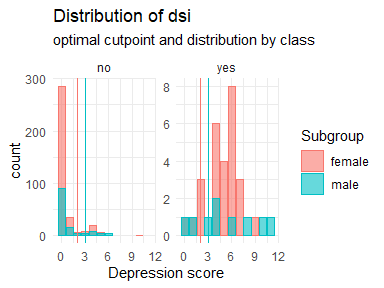## Flexible plotting function

Using plot_cutpointr any metric can be chosen to be plotted on the x- or y-axis and results of cutpointr() as well as roc() can be plotted. If a cutpointr object is to be plotted, it is thus irrelevant which metric function was chosen for cutpoint estimation. Any metric that can be calculated based on the ROC curve can be subsequently plotted as only the true / false positives / negatives over all cutpoints are needed. That way, not only the above plots can be produced, but also any combination of two metrics (or metric functions) and / or cutpoints. The built-in metric functions as well as user-defined functions or anonymous functions can be supplied to xvar and yvar. If bootstrapping was run, confidence intervals can be plotted around the y-variable. This is especially useful if the cutpoints, available in the cutpoints function, are placed on the x-axis. Note that confidence intervals can only be correctly plotted if the values of xvar are constant across bootstrap samples. For example, confidence intervals for TPR by FPR (a ROC curve) cannot be plotted easily, as the values of the false positive rate vary per bootstrap sample.

set.seed(1234)
opt_cut_b <- cutpointr(suicide, dsi, suicide, boot_runs = 500)
## Assuming the positive class is yes
## Assuming the positive class has higher x values
## Running bootstrap...
plot_cutpointr(opt_cut_b, xvar = cutpoints, yvar = sum_sens_spec, conf_lvl = 0.9)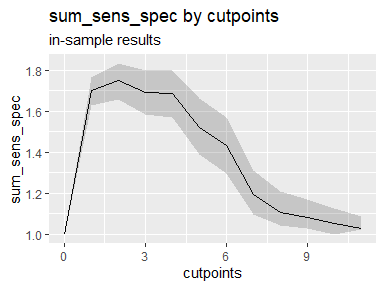plot_cutpointr(opt_cut_b, xvar = fpr, yvar = tpr, aspect_ratio = 1, conf_lvl = 0)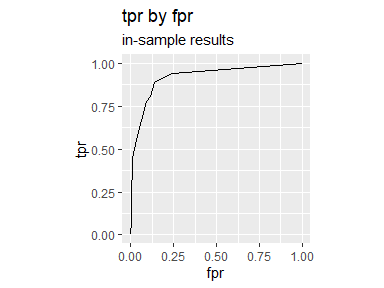plot_cutpointr(opt_cut_b, xvar = cutpoint, yvar = tp, conf_lvl = 0.9) +
geom_point()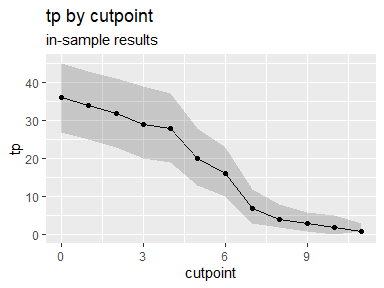## Manual plotting

Since cutpointr returns a data.frame with the original data, bootstrap results, and the ROC curve in nested tibbles, these data can be conveniently extracted and plotted manually. The relevant nested tibbles are in the columns data, roc_curve and boot. The following is an example of accessing and plotting the grouped data.

library(dplyr)
library(tidyr)
opt_cut_b_g |>
select(data, subgroup) |>
unnest(cols = data) |>
ggplot(aes(x = suicide, y = dsi)) +
geom_boxplot(alpha = 0.3) +
facet_grid(~subgroup)